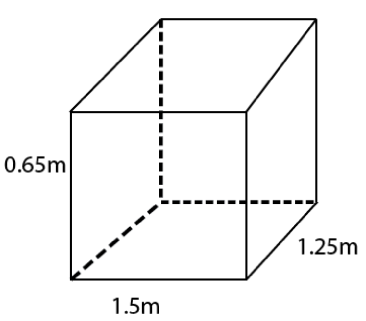# A plastic box 1.5 m long, 1.25 m wide and 65 cm deep, is to be made. It is to be open at the top. Ignoring the thickness of the plastic sheet, determine: (i) The area of the sheet required for making the box. (ii) The cost of sheet for it, if a sheet measuring 1m2 costs Rs. 20.

Given

Length of box (l) = 1.5 m

Breadth of box (b) = 1.25 m

Height of box (h) = 65 cm = 0.65 m

The box is open at top

Solution

(i) The area of the sheet required for making the box.Therefore, area of sheet required = lb + 2lh + 2bh

= (1.5 * 1.25 + 2 * 1.5 * 0.65 + 2 * 1.25 * 0.65)m2

= (1.875 + 1.95 + 1.625)m2

= 5.45 m

(ii) The cost of the sheet for it, if a sheet measuring 1m2 costs 20 rupees.
Solution:

The area of the box is 5.45 m2

Given, 1 m2 costs 20 rupees

Therefore, cost of 5.45 m2 box is = (5.45 * 20) rupees = 109 rupees